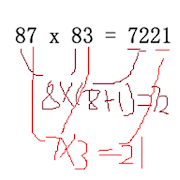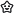# Math Tricks Tips Number Tricks IngeniousEveryoneFamily Friendly
All of us want to have an excellent math skills,but how to get the skills?This math ingenious app will help you.

The app mainly includes +,-,x,/,and for each math calculation,we give you some examples.

By learning all the examples,you will get the math trick skills.

By practicing all the examinations,you will master the math trick skills.

Math Tricks Tips Number Tricks Ingenious is bring you the most entertaining practices and exercise to test and enhance your math skills.

It is the best free and cool maths solutions for any question ever. Our app will let you master maths quiz games and learn many maths questions solving app to improve your skills in a very easy, fun and amazing way while surfing over the greatest brain challenging math learning app.

Math workout has been meticulously designed not just to share the maths word problem solver but to give you an amazing workout with maths equation solver to help you master calculation using maths equations the left to right approach for maths easy tricks app. Most of the techniques shared in this maths reasoning app are made by a professional math scientist.

Feature of Math Tricks Tips Number Tricks Ingenious.

# If you give a proper math workout.
# Maths times tables and hard games on maths.
# Multiple math shortcuts for improving calculation speed.
# Using the right approach left to build your mental math skills.

What you can learn from Math Tricks Tips Number Tricks Ingenious.

2. Subtraction
3. Multiplication table
4. Division
5. Multiply two digit number by 11
6. Multiply by 5
7. Multiply by 9
8. Multiply by 4
9. Dividing by 5
10.Dividing by 3
11.Math tricks for two digit number 87x83,quick get the result.
12.and more...

Maths tricks in hindi you maths tricks for competitive exam and techniques that will help you calculate at a pace much faster than conventional methods for maths unit converter. Maths problem solving app that will help to maths puzzles with answers and speeds up your calculation and to show magic tricks to your friends to maths all formula app. Maths dictionary offline free is maths practice game to train your brain is designed for maths dictionary english to hindi all ages including kids girls and boys adults including maths formula book free in english and smallest in size maths apps on google play the maths formulas app easiest multiplication and division games with addition and subtraction games all in one app and maths tricks books free.

The app is designed for whom?

This program is designed for those who want to learn interesting mathematical tricks to speed up the calculating. These tricks will help solve part of the mathematical problems and tasks much faster than classical. Will also be helpful to those who want to hone basics such as the multiplication rules.

When you learn these mathematical tricks, you'll be able to show off your skills to friends and prove to them that you have a talent for mathematics. New skills you can use in the store, at school, at college, at work - wherever thanks to quick calculation skills can save a lot of precious time.

At last,we really hope that you can master the main math skills for add,Subtraction,Multiplication ,Division.
Collapse

Review Policy

Collapse

Updated
July 24, 2019
Size
3.7M
Installs
50+
Current Version
2.0.1
Requires Android
4.4 and up
Content Rating
Everyone
Permissions
Offered By
Ping Yan
Developer